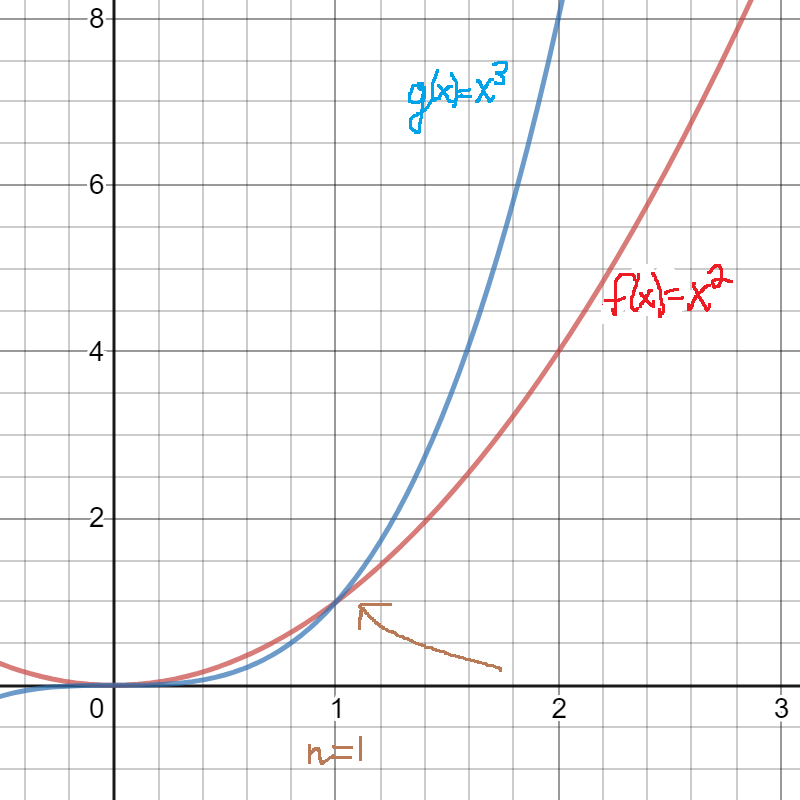$$\newcommand{\id}{\mathrm{id}}$$ $$\newcommand{\Span}{\mathrm{span}}$$ $$\newcommand{\kernel}{\mathrm{null}\,}$$ $$\newcommand{\range}{\mathrm{range}\,}$$ $$\newcommand{\RealPart}{\mathrm{Re}}$$ $$\newcommand{\ImaginaryPart}{\mathrm{Im}}$$ $$\newcommand{\Argument}{\mathrm{Arg}}$$ $$\newcommand{\norm}{\| #1 \|}$$ $$\newcommand{\inner}{\langle #1, #2 \rangle}$$ $$\newcommand{\Span}{\mathrm{span}}$$

# 8.1: Big O

$$\newcommand{\vecs}{\overset { \rightharpoonup} {\mathbf{#1}} }$$ $$\newcommand{\vecd}{\overset{-\!-\!\rightharpoonup}{\vphantom{a}\smash {#1}}}$$$$\newcommand{\id}{\mathrm{id}}$$ $$\newcommand{\Span}{\mathrm{span}}$$ $$\newcommand{\kernel}{\mathrm{null}\,}$$ $$\newcommand{\range}{\mathrm{range}\,}$$ $$\newcommand{\RealPart}{\mathrm{Re}}$$ $$\newcommand{\ImaginaryPart}{\mathrm{Im}}$$ $$\newcommand{\Argument}{\mathrm{Arg}}$$ $$\newcommand{\norm}{\| #1 \|}$$ $$\newcommand{\inner}{\langle #1, #2 \rangle}$$ $$\newcommand{\Span}{\mathrm{span}}$$ $$\newcommand{\id}{\mathrm{id}}$$ $$\newcommand{\Span}{\mathrm{span}}$$ $$\newcommand{\kernel}{\mathrm{null}\,}$$ $$\newcommand{\range}{\mathrm{range}\,}$$ $$\newcommand{\RealPart}{\mathrm{Re}}$$ $$\newcommand{\ImaginaryPart}{\mathrm{Im}}$$ $$\newcommand{\Argument}{\mathrm{Arg}}$$ $$\newcommand{\norm}{\| #1 \|}$$ $$\newcommand{\inner}{\langle #1, #2 \rangle}$$ $$\newcommand{\Span}{\mathrm{span}}$$

## Big O

The idea of Big O is to characterize functions according to their growth rates. The O refers to the order of a function. In computer science, Big O is used to classify algorithms for their running time or space requirements.

Notice in the figure below that $$f(x)>g(x)$$ right before $$x=1$$.  However, for $$x>1$$, we see that $$g(x)>f(x).$$  In the long run (namely after $$x>1$$) $$g(x)$$ overtakes $$f(x).$$

We say "$$f$$ is of order at most $$g$$" or "$$f(x)$$ is Big O of $$g(x)$$" .

We write:                $$f(x)= O\big(g(x)\big).$$In our definition of Big O notation, there are certain parameters.

• We use $$x$$ greater than a certain initial value, $$n$$; in the diagram above, $$n=1$$.
• We use absolute value for both functions.
• We use $$k$$ as a constant multiplied by the function inside the O.

### Definition: Big O Notation

$f(x)= O\big(g(x)\big).$

if and only if           there exist real numbers $$k,n$$ with $$k>0 , n\geq 0$$ such that

$|f(x)| \leq k|g(x)| \qquad \forall x>n.$

Example $$\PageIndex{1}$$

Take this statement and express it in Big O notation:  $$|7x^5+4x^3+x| \leq 14|x^5|$$  for $$x>1.$$

Solution

$$(7x^5+4x^3+x) \mbox{ is } O(x^5)$$

### Comparing orders of common functions

A constant function, such as $$f(x)=6$$ does not grow at all.  Logarithmic functions grow very slowly. Here is a list of some common functions in increasing order of growth rates.

constant function, logarithmic function, polynomial function, exponential function

Example $$\PageIndex{2}$$

Put these functions in order of increasing growth rates:

$\log_6x,\qquad x^5,\qquad 2^x,\qquad x^2,\qquad \log_{15}x,\qquad 100x^4,\qquad 64x+1000,\qquad x^5 \log_6x,\qquad 5^x, \qquad 6$

Solution

$6, \qquad \log_6x,\qquad \log_{15}x,\qquad 64x+1000,\qquad x^2,\qquad 100x^4,\qquad x^5,\qquad x^5 \log_6x, \qquad 2^x,\qquad 5^x$

## Proofs

We will be using the Triangle Inequality Theorem which is $|x+y|\ \leq |x|+|y|.$

Example $$\PageIndex{3}$$

Prove: $$4x^3-11x^2+3x-2=O(x^3)$$

Proof

Choose $$n=1$$, i.e. $$x \geq 1.$$
$$|4x^3-11x^2+3x-2 |\leq |4x^3|+|-11x^2|+|3x|+|-2| \qquad \mbox{ by the Triangle Inequality Theorem}$$
$$\qquad \qquad \qquad \qquad \qquad=4x^3+11x^2+3x+2 \qquad \mbox{ applying absolute value; note: }x \mbox{ is positive}$$
$$\qquad \qquad \qquad \qquad \qquad\leq 4x^3+11x^3+3x^3+2x^3 \qquad \mbox{ since }x \mbox{ is positive and greater than 1}$$
$$\qquad \qquad \qquad \qquad \qquad =20x^3$$
$$\qquad \qquad \qquad \qquad \qquad=20|x^3|\qquad \mbox{ since }x \mbox{ is positive and greater than 1}$$

Thus  for all $$x \geq 1,$$ $$|4x^3-11x^2+3x-2 | \leq 20|x^3|$$

Therefore, using $$n=1$$ and $$k=20$$,  $$4x^3-11x^2+3x-2=O(x^3)$$  by the definition of Big O.

## Summary and Review

• Big O is used to compare the growth rates of functions.
• Be sure to understand the examples here.

## Exercises

exercise $$\PageIndex{1}$$

True or False?

(a) $$11x^3=O(87x^2)$$

(b) $$x^{13}=O(3^x)$$

(c) $$-2x=O(58\log_{35}x)$$

(a) false
(b) true
(c) false

Exercise $$\PageIndex{2}$$

True or False?

(a) $$4x^3+12x^2+36=O(x^3)$$

(b) $$.01x^5=O(48x^4)$$

(c) $$4^x=O(x^7)$$

(d) $$3x\log_2x=O(25x)$$

Exercise $$\PageIndex{3}$$

True or False?

(a) $$23\ln x=O(3x)$$

(b) $$7x^5=O(x^5)$$

(c) $$x^5=O(7x^5)$$

all true

Exercise $$\PageIndex{4}$$

Prove: $$2x^5+3x^4-x^3+5x=O(x^5)$$

Example $$\PageIndex{5}$$

Put these functions in order of increasing growth rates:

$x^7,\qquad 6^x,\qquad 78x^2,\qquad x^2\log x,\qquad 1000x,\qquad 7, \qquad \log_{11}x$

$7, \qquad \log_{11}x, \qquad 1000x, \qquad 78x^2,\qquad x^2\log x, \qquad x^7,\qquad 6^x$
Exercise $$\PageIndex{6}$$
Take this statement and express it in Big O notation:  $$|2x^4-5x^3+x^2-5| \leq 13|x^4|$$  for $$x>1.$$